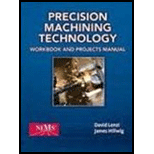Chapter 6.3, Problem 5RQ### Precision Machining Technology (Mi...

2nd Edition
Peter J. Hoffman + 2 others
ISBN: 9781285444543

#### Solutions

Chapter
Section### Precision Machining Technology (Mi...

2nd Edition
Peter J. Hoffman + 2 others
ISBN: 9781285444543
Textbook Problem

# Calculate spindle speed and feed for the two following situations: a. Use an HSS 1/2"-diameter three-flute endmill to mill 6061 aluminum 350 SFPM using a 0.0015" chip load. b. Use a carbide 3"-diameter eight-tooth shell mill to machine H13 tool steel at 200 SFPM using a 0.003" chip load.

(a)

To determine

The spindle speed for milling operation.

The feed rate for milling operation.

Explanation

Calculate the spindle speed for milling operations.

RPM=3.82×CSD (I)

Here, the spindle speed in rpm is RPM, the cutting speed of the tool is CS and the diameter of the cutting tool is D.

Calculate the feed rate for milling operations

IPM=FPT×N×RPM (II)

Here, the feed per tooth is FPT and the number of flute is N.

Conclusion:

Substitute 350 for CS and 1/2" for D in Equation (I).

RPM=3

(b)

To determine

The spindle speed for milling operation.

The feed rate for milling operation.

### Still sussing out bartleby?

Check out a sample textbook solution.

See a sample solution

#### The Solution to Your Study Problems

Bartleby provides explanations to thousands of textbook problems written by our experts, many with advanced degrees!

Get Started

## Additional Engineering Solutions

#### Find more solutions based on key concepts